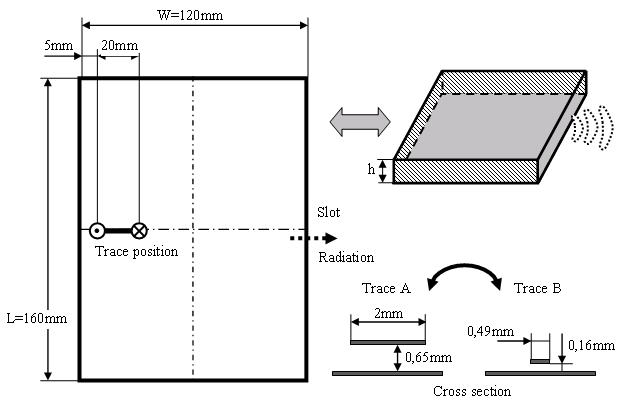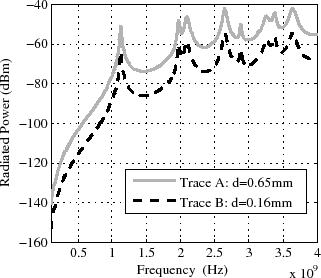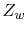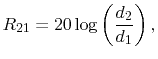# 8.4 Rule 4: Reduction of the trace height d above the ground plane reduces EMI(a) Placement of Trace A and Trace B.(b) Radiated power. Width of Trace B: b=0.49mm. (c) Radiated power. Width of Trace B: b=2mm.
Figure 8.1: The trace width is 0.2mm, the trace height above the ground plane is 0.65mm. A 10mV voltage source with an impedance equal to the characteristic impedance of the tracedrives the trace which is terminated with a 10pF capacitance.

With reduction of the trace height above the PCB ground plane, the radiated emission is reduced in the whole frequency band by the factor(8.12)
where d1 denotes the initial trace height above the ground plane and d2 denotes the new, reduced trace height above the ground plane. This reduction can only be realized, when the trace width is also reduced, to conserve the characteristic impedance of the trace Zw. Figure 8.7(b) shows the emission reduction of about 12dB when the trace width is correctly reduced. A reduction of the trace height above the ground plane without reduction of the trace width leads to a reduction of Zw through the increased capacitance. This will increase the trace currents, which almost compensates the emission reduction effect from the height reduction, as illustrated in Figure 8.7(c). The reduction of the trace height above the ground plane can be realized by using thinner dielectric layers for the PCB. A PCB layer thickness change has has an influence on every circuit net, which is routed on the copper layers around the changed dielectric layer. Thus, a dielectric layer thickness change must be carried out very carefully. A correct characteristic impedance of a trace, which matches the termination impedances is crucial for all high speed signal traces which are not electrically short, in order to avoid reflections and ensure signal integrity. Therefore, the design of these signal nets has to be checked and eventually adapted at any thickness change of PCB layers. The power distribution network on the PCB also has to be checked and eventually changed to ensure power integrity.

C. Poschalko: The Simulation of Emission from Printed Circuit Boards under a Metallic Cover